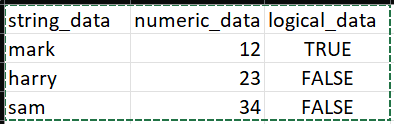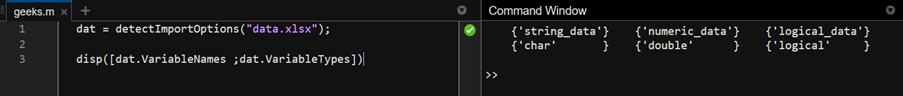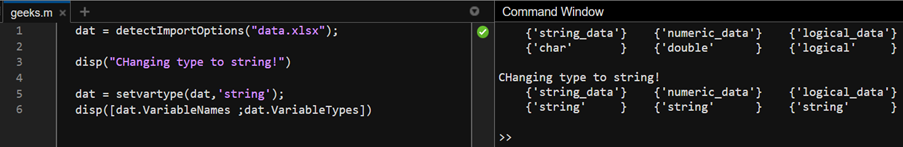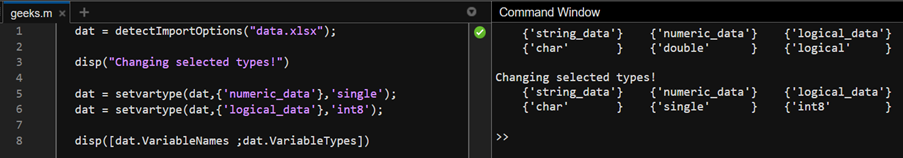Open in App
Not now

# Set Variable Data Types in MATLAB

• Last Updated : 12 Dec, 2022

There are many cases when a user has to import data into MATLAB script from various files. These file types could be .txt, .xlsx, .csv, .dat, etc. types. Now, each file type has its own method of defining data types. However, for computation purposes, MATLAB requires the data to be of numeric type, rather than character. Thus, there is a requirement to set the data types of these imported data. MATLAB solves this problem by providing the setvartype function to change the data type of a given variable from the imported data. In this article, we shall see the usage of the same function with the help of some examples.

### Syntax:

<list_of_variables> = setvartype(<list_of_variables>, selection, type)

The first argument to the function is the list of imported data variables. The selection arguments allows setting the data type of selected variables from list_of_variables. Lastly, the type argument takes the data type to be set for the selected variables. If the selection argument is omitted then, setvartype changes the data type of all variables passed in the list.

## Example 1:

We shall import the following data into our MATLAB script to illustrate the usage of setvartype.To import this excel spreadsheet, we shall use the detectImportOptions function.

## Matlab

 `% MATLAB Code``dat = detectImportOptions(``"data.xlsx"``);`` ` `% Displaying the variable names and ``% their data type using the dat object``disp([dat.VariableNames ;dat.VariableTypes])`

Output:

Above code imports the data.xlsx file and displays its variable names and their types.As can be seen that first variable is of character type, the second one is of double and last one is a logical type. Now we shall change all three of these variables to string type using the setvartype function.

## Matlab

 `% MATLAB Code``dat = detectImportOptions(``"data.xlsx"``);``disp(``"Changing type to string!"``)`` ` `% Changing all variables to string type``dat = setvartype(dat,``'string'``);``disp([dat.VariableNames ;dat.VariableTypes])`

Output:

As there is no selection of variables made in the above code, setvartype will change all the variables in dat.## Example 2

Now we shall use the same initial data file and change the data type of selected variables by passing the optional selection parameter.

## Matlab

 `% MATLAB Code``dat = detectImportOptions(``"data.xlsx"``);``disp(``"Changing selected types!"``)`` ` `% Changing numeric_data to single type``dat = setvartype(dat,{``'numeric_data'``},``'single'``);`` ` `% Changing numeric_data to 8-bit integer``dat = setvartype(dat,{``'logical_data'``},``'int8'``);`` ` `disp([dat.VariableNames ;dat.VariableTypes])`

Output:

In the above code, we change the ‘numeric_data’ to single and the ‘logical_data’ to int8 type as it contains Boolean data. The output will be:My Personal Notes arrow_drop_up